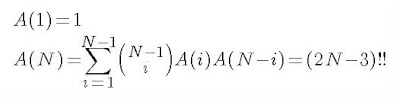## Tuesday, August 10, 2010

### Combinatorial Madness - The Final Post

So, a colleague of mine was able to solve the original potions problem such that the solution was the double factorial and not the summation. Thus, he was able to kill two birds with one stone. His way of looking at the problem shows both that the solution of the problem is, in fact, the double factorial and that by doing so, produces a proof by combinatorial argument of the double factorial identity.

For those unfamiliar, a proof by combinatorial argument is basically where you show two different ways to count the same thing, each having its own expression. Thus, both expressions must be equal, since they both count the same thing.

I take no credit for the following... it was solely the work of my colleague. If I mangle his argument, I apologize. I'm sure that I could be clearer about some parts, but I do think that the reasoning is solid. Anyway, this is basically how it goes.

Say that we want to know the solution to the N potions problem. Suppose that the solution of the k potions problem is A(k). With (N-1) potions, the total number of final potion products is A(N-1). Now, in producing a single final product with (N-1) initial potions, we would have gone through (N-2) iterations where some potion was combined with another potion in each iteration.

So, we can view these (N-2) combination actions as C_1, C_2, C_3, ..., C_(N-2). Any one of these combinations can be expressed as X+Y, X and Y being available potions at that junction. In order to tackle the N potion problem, we would like to add a new potion (the Nth potion -- call it Z) to the total mix. The question now is how can we add it such that we don't end up with any overcounting, etc?

For any combination action, we can inject the newly introduced Nth potion (Z) into that particular iteration -- however, notice that there are two ways in which this injection can happen. We can replace the combination that we're focused on with one where we've injected Z as ((X + Z)+Y) or as (X + (Y+Z)). Since there are (N-2) combination actions where we can perform this injection, we have 2(N-2) possible injection points.

We're missing one more injection point. That is the case where we simply combine the newly introduced Nth potion at the very end after C_(N-2) has produced some final product. There is exactly one injection point at the very end of our chain of C_k's. That adds one, so that gives us (2(N-2) + 1) injection points, and it's clear that adding Z to each of these injection points creates a completely new final product given this particular chain of combination actions (C_k's).

Now, we also know that there are A(N-1) different chains of combination actions that produce unique final products. Thus, we have (2(N-2)+1) * A(N-1) total final products for the N potions case. This simplifies to (2N-3) * A(N-1), which is clearly equivalent to (2N-3)!!, especially given that we know A(2) = 1 (the trivial 2-potion problem) .

So, this is a direct explanation of why the N-potion problem solution is (2N-3)!!, and it gives us the combinatorial proof that (2N-3)!! is equal to our other expression (from the previous post):I know the above wasn't explained previously. That was me being lazy. Anyway, the explanation of why the above summation counts the final products in the N-potion problem is as follows (this one I actually helped come up with).

We are essentially enumerating all possible 2-partitions of the N potions being combined. A(i) represents the total final product count of the first of two partitions where the Nth potion is not a member. The A(N-i) represents the total final product count of the second of the two partitions and it is where the Nth potion is a member. The product of the two final product counts (i.e. A(i) * A(N-i) will result in the total number of final products when all N potions (both partitions) are considered). We are allowed to do this since our construction ensures no overcounting.

To further clarify, our loop going from i=1 to (N-1) gives us all the possible cardinalities of our partitions. We must multiply by ((N-1) Choose i) in order to produce all the combinations possible that make up the partitions with those cardinalities. Again, since we cannot overcount due to our construction, we can now sum the final product counts for all the possible partitions, giving us A(N).

Hope that was clear enough. And, in a way it was nice that we did not initially come up with the solution that leads directly to the double factorial. Because of this, we now have a pretty nifty combinatorial identity relating the recursive summation described above to the double factorial.